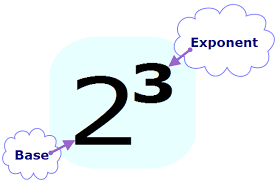Basic Exponents
4 years ago
greed123
Save
Edit
Host a game
Live GameLive
Homework
Solo Practice
Practice20 QuestionsShow answers
• Question 1
30 seconds
Q. Write each product using an exponent.
4 x 4 x 4 x 4 x 4
45
54
46
46
• Question 2
30 seconds
Q. Write each product using an exponent.
7 x 7 x 7 x 7 x 7 x 7 x 7 x 7
77
78
87
79
• Question 3
120 seconds
Q. Which is equivalent to 24
8
20
16
12
• Question 4
120 seconds
Q. Which is equivalent to 5 x 5 x 5 x 5
53
54
45
55
• Question 5
120 seconds
Q. Which is equivalent to 9o
1
0
9
• Question 6
120 seconds
Q. What is equivalent to 5 squared?
25
10
55
30
• Question 7
120 seconds
Q. How do you correctly say 43
4 squared
4 cubed
4 to the power of 2
• Question 8
120 seconds
Q. Which is equivalent to 34
27
9
81
12
• Question 9
30 seconds
Q. What is another way to write 0.35 × 0.35 × 0.35 × 0.35 × 0.35 × 0.35?
6 × 0.35
0.356
60.35
6 + 0.35
• Question 10
30 seconds
Q. What is another way to write 63?
6 × 6 × 6
3 × 3 × 3 × 3 × 3 × 3
6 + 6 + 6
6 × 3
• Question 11
30 seconds
Q. What is another way to write 7 cubed?
7 × 7 × 7
3 × 3 × 3 × 3 × 3 × 3 × 3
21
7 + 3
• Question 12
30 seconds
Q. What is the value of 43?
12
7
64
16
• Question 13
30 seconds
Q. What is the value of 25?
32
16
10
8
• Question 14
30 seconds
Q. Write each product using an exponent.
14 x 14
214
142
14
1414
• Question 15
60 seconds
Q. What is the BASE in the power below?
43
4
3
43
64
• Question 16
60 seconds
Q. What is the Exponent in the following example?
-25
-2
2
5
-25
• Question 17
60 seconds
Q. Another name for exponent is _______________ .
base
power
fractional
• Question 18
60 seconds
Q. What is another way to write x4?
x ∗ 4
4 ∗ 4 ∗ 4 ∗ 4 ∗ 4
x  ∗  x ∗  x  ∗  x
x + x + x + x
• Question 19
60 seconds
Q. 40
0
1
4
-4
• Question 20
180 seconds
Q. What is the missing exponent in the equation 2? = 16?